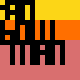# Processing Month, Day 3 - Triangle Grids

Posted by Jan Vantomme on 03 May 2011.
Tags:

Day three will be all about triangles. But we’re not going to use the `triangle()` function. We will draw points and lines again just like the examples from day one and two, but we will restrict the lines so they can only be drawn on a grid based on regular triangles. To make it a little more interesting, we’ll also add animation.

The starting point for the sketch will be located at the center of the window. Since we are going to animate the line, we’ll need to keep track of the location of the current point and the previous point so we can draw a line between them. We also need a radius to calculate new points. So you need to declare these variables at the beginning of your sketch.

``` float radius = 20;float x, y;float prevX, prevY;  ```

Next thing we need to do is give these variables a value. We want to start at the center of the window so we’ll calculate those x and y values by dividing the `width` and `height` variables with two. These built-in system variables are set with the `size()` function and are always available to use in your sketch.

``` x = width / 2;y = height / 2;    prevX = x;prevY = y;  ```

For the next part, we’ll move to the `draw()` method. To calculate the next point we’ll use `cos()` and `sin()` like we did on day one. We are going to use regular triangles so our line can move in six fixed directions. The algorithm is easy:

1. The sum of the three angles in a triangle is 180 degrees or PI radians.
2. Since we use a regular triangle, they are all equal so their value is `180 / 3 = 60` degrees.
3. A circle is 360 degrees or TWO_PI radians. If we divide this by 60, we’ll get 6 possible directions.
4. The angles for those directions are 0, 60, 120, 180, 240 and 300.

I want the computer to choose which direction to draw, so I’ll use a random number to calculate the direction. The problem is that the `random()` function generates floats, and I want the result to be one of the integers 0, 1, 2, 3, 4 or 5 to calculate the right angle. This can be fixed by using the `floor()` function. This will round the random number between 0 and 6 down to the closest integer value, so we’ll end up with the numbers we want.

``` float angle = (TWO_PI / 6) * floor( random( 6 ));x += cos( angle ) * radius;y += sin( angle ) * radius;  ```

This will move the point to a new place on the grid each time the `draw()` method is called. Next thing we need to do is draw a line between the current point and the previous point. We also need to set the coordinates for the previous point to those of the current point at the end of `draw()`. Otherwise there wouldn’t be any animation after the first frame.

``` stroke( 255, 64 );strokeWeight( 1 );line( x, y, prevX, prevY );strokeWeight( 3 );point( x, y );// update prevX and prevY with the new valuesprevX = x;prevY = y;  ```

If you run the sketch, you’ll see that the line might leave the screen and never return. We need to implement a little algorithm right after the new x and y values are calculated to make sure that the line doesn’t leave the screen. We can do this by checking if the new x value is lower than 0 or higher than the width of the sketch. If this is the case, we’ll set the x and y value to their previous values again, so the line won’t leave the screen. Same thing can be done for the y value.

``` if ( x < 0 || x > width ) {    x = prevX;    y = prevY;}if ( y < 0 || y > height) {    x = prevX;    y = prevY;}  ```

To make our little sketch a little more interesting, we’ll fade the background so our line moves like a snake over the screen. We’ll also add the option to toggle the fading by pressing a key on the keyboard. To do this we need to declare a boolean at the beginning of the sketch.

``` Boolean fade = true;  ```

The next piace of code goes right at the beginning of the `draw()` method. We first need to check if the value of fade is true. If this is the case, the block of code within the if-statement will draw a transparent black rectangle on top of everything.

``` if (fade) {    noStroke();    fill( 0, 4 );    rect( 0, 0, width, height );}  ```

To toggle the fading, we need to add the keyPressed() method to our sketch. This function is called each time a key on the keyboard is pressed. We need to check if that key was the letter f and change the value of the fade variable.

``` void keyPressed(){    if (key == 'f') {        fade = !fade;    }}  ```

Run the sketch and you’ll see that the older parts of our continuous line fade away in the background. Try pressing the letter f to disable or enable the fading.

### Examples